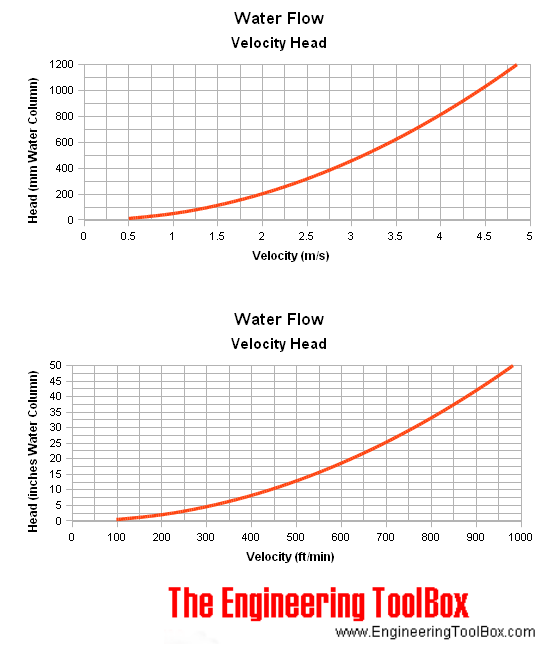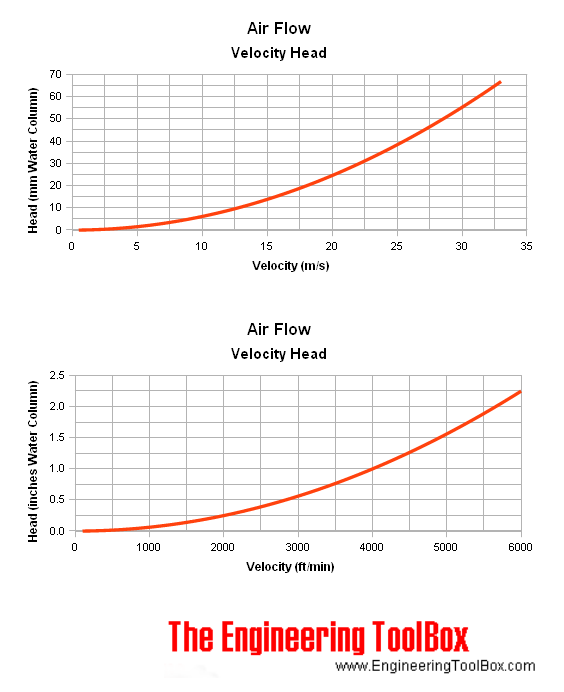Engineering ToolBox - Resources, Tools and Basic Information for Engineering and Design of Technical Applications!

## Dynamic pressure or velocity head.

The dynamic pressure in a fluid flow can be expressed as

p d = 1/2 ρ f v 2 (1)

where

p d = dynamic pressure in fluid (Pa, ps f (lb/ft 2 ))

ρ f = density of fluid (kg/m 3 , slugs /ft 3 )

v = fluid velocity (m/s, ft/s)

• 1 Pa = 10 -6 N/mm 2 = 10 -5 bar = 0.1020 kp/m 2 = 1.02x10 -4 m H 2 O = 9.869x10 -6 atm = 1.45x10 -4 psi (lbf/in 2 )
• 1 psf (lb f /ft 2 ) = 47.88 N/m 2 (Pa) = 0.006944 lb f /in 2 (psi)

Head loss as water column can be calculated by dividing the dynamic pressure with the specific weight of water like

h w = p d / γ w

= ( 1/2 ρ f v 2 ) / ( ρ w g)

= ρ f v 2 / (2 ρ w g) (2)

where

h w = head water (m Water, ft Water)

γ w = ρ w g = specific weight water (9807 N/m 3 , 62.4 l b f /ft 3 )

where

ρ w = density of water (1000 kg/m 3 , 1.940 slugs/ft 3 )

g = acceleration of gravity ( 9.81 m/s 2 , 32.174 ft/s 2 )

### Water Flow

If the flowing fluid is water - then ρ f = ρ w , and (2) can be modified to

h w = v 2 / 2 g (3)

Or simplified to

#### Metric Units

h w (m H2O) = 0.051 v 2 (3b)

h w (mm H2O) = 51 v 2 (3c)

where

v = velocity (m/s)

#### Imperial Units

h w (ft H2O) = 0.0155 v fps 2 (3d)

h w (in H2O) = 0.186 v fps 2 (3e)

where

v fps = velocity (ft/s)

or

h w (ft H2O) = 0.0155 (v fpm / 60) 2 (3f)

h w (in H2O) = 0.186 (v fpm / 60) 2 (3g)

where

v fpm = velocity (ft/min)

#### Water Flow - Head to Velocity Calculator

This calculator can be used to calculate water velocity in a pipe or water stream from a measured head of water column. The dynamic pressure as head is typically measured with a pitot tube .

#### Water Flow - Velocity and Dynamic Head Chart#### Water Flow - Velocity Heads

Velocity Heads vs. water Flow Velocities
Water Flow Velocity
- v -
(ft/sec)

- h w -
(ft H2O)

0.5 0.004
1.0 0.016
1.5 0.035
2.0 0.062
2.5 0.097
3.0 0.140
3.5 0.190
4.0 0.248
4.5 0.314
5.0 0.389
5.5 0.470
6.0 0.560
6.5 0.657
7.0 0.762
7.5 0.875
8.0 0.995
8.5 1.123
9.0 1.259
9.5 1.403
10.0 1.555
11.0 1.881
12.0 2.239
13.0 2.627
14.0 3.047
15.0 3.498
16.0 3.980
17.0 4.493
18.0 5.037
19.0 5.613
20.0 6.219
21.0 6.856
22.0 7.525
• 1 ft (foot) = 0.3048 m = 12 inches = 0.3333 yd

### Air Flow

#### Metric Units

If the flowing fluid is air with ρ f = 1.205 kg/m 3 (20 o C) and the reference fluid is water with ρ w = 1000 kg/m 3 - (2) can be simplified to

h w (m H2O) = ((1.205 kg/m 3 ) v 2 ) / (2 ( 1000 kg/m 3 ) 9.81 m/s 2 )

= 6.1 10 -5 v 2 (4)

where

v = air velocity (m/s)

or

h w (mm H2O) = 6.1 10 -2 v 2 (4b)

Note! - these simplified equations must be relaculated for other air temperatures and densities .

#### Imperial Units

h w (ft H2O) = (v / C) 2 / 12                                   (4c)

h w (in H2O) = (v / C) 2 (4c)

where

v = velocity (ft/min)

C = constant (4005 for temperature 70 o F)

The constant C and temperature:

 Air Temperature ( o F) C 35 3872 40 3891 45 3910 50 3932 55 3952 60 3970 65 3987 70 4005 75 4025 80 4045 85 4064 90 4081 95 4101 100 4117 150 4298

#### Air Flow - Head to Velocity Calculator

This calculator can be used to calculate air velocity in a duct or air stream from a measured head of water column. The dynamic pressure as head is typically measured with a pitot tube .

#### Velocity and Dynamic Head Chart

Charts are based on air density 1.205 kg/m 3 and water density 1000 kg/m 3 .#### Air Flow - Velocity Heads

Table based on ρ f = 1.205 kg/m 3 (C = 4005, temperature 70 o F) and ρ w = 1000 kg/m 3 ( 1.940 slugs/ft 3 ) .

Velocity Heads vs. Air Flow Velocities
Air Flow Velocity
- v -
(ft/sec)

- hw -
(ft H2O)

5 0.00047
10 0.0019
15 0.0042
20 0.0075
25 0.012
30 0.017
35 0.023
40 0.030
45 0.038
50 0.047
55 0.057
60 0.067
65 0.079
70 0.092
75 0.11
80 0.12
85 0.14
90 0.15
95 0.17
100 0.19

## Related Topics

• ### Fluid Mechanics

The study of fluids - liquids and gases. Involving velocity, pressure, density and temperature as functions of space and time.

## Related Documents

• ### Dynamic Pressure

Dynamic pressure is the kinetic energy per unit volume of a fluid in movement.
• ### Pipe and Duct Systems - Total Head Loss

Major and minor loss in pipes, tubes and duct systems.
• ### Pipe Line Velocities vs. Fluid

Typical fluid flow velocities for common liquids, gases and vapors.
• ### Pitot Tubes

Pitot tubes can be used to measure fluid flow velocities by measuring the difference between static and dynamic pressure in the flow.
• ### Static Pressure vs. Head

Static pressure vs. pressure head in fluids.
• ### Velocity-Area Flowmetering

Flow rate or discharge in an open conduit, channel or river can be calculated with the velocity-area principle.

## Search

Search is the most efficient way to navigate the Engineering ToolBox.

## Engineering ToolBox - SketchUp Extension - Online 3D modeling!

Add standard and customized parametric components - like flange beams, lumbers, piping, stairs and more - to your Sketchup model with the Engineering ToolBox - SketchUp Extension - enabled for use with older versions of the amazing SketchUp Make and the newer "up to date" SketchUp Pro . Add the Engineering ToolBox extension to your SketchUp Make/Pro from the Extension Warehouse !

We don't collect information from our users. More about

## Citation

• The Engineering ToolBox (2005). Velocity Pressure Head. [online] Available at: https://www.engineeringtoolbox.com/velocity-head-d_916.html [Accessed Day Month Year].

Modify the access date according your visit.

11.23.12